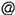Jehanzeb H. Chaudhry

Contact
jehanzebunmedu

Dept of Mathematics and Statistics
University of New Mexico
310 SMLC
Albuquerque, NM, 87131

I am an associate professor in the Mathematics and Statistics Department at the University of New Mexico. My research interests are in the design and analysis of numerical methods for partial differential equations. In particular my research involves

• Uncertainty quantification
• Parallel-in-time integration
• Implicit-Explicit (IMEX) time integration
• Least-squares finite element methods
• Poisson-Boltzmann and Poisson-Nernst-Planck equations